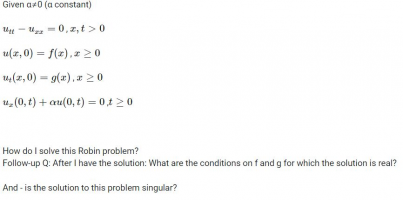# PDE: first quadrant robin wave equation - homogenous

#### Subhotosh Khan

##### Super Moderator
Staff member
I started by solving like I would do with any wave equation but couldn't understand how to incorporate the Robin IC.
got stuck!
could really use your help!This almost looks like equation of heat transfer in a plate. I have NOT solved this problem - but I would start with the assumption:

u (x, t) = P(x) * Q(t)

Please show us what you have tried and exactly where you are stuck.

Please follow the rules of posting in this forum, as enunciated at:

•topsquark

#### HALEY PHAM

##### New member
I happened to forget to add the c^2 in the first equation indicating this is in fact a wave equation.
I started by solving like I would do with any wave equation (and got to the left and right mover F(x), G(x) - am I supposed to sum them and get the d'Alembert formula?)
but couldn't understand how to incorporate the Robin boundary conditions.

#### Subhotosh Khan

##### Super Moderator
Staff member
I happened to forget to add the c^2 in the first equation indicating this is in fact a wave equation.

So post the corrected equation after inserting c^2.

I started by solving like I would do with any wave equation (and got to the left and right mover F(x), G(x) - am I supposed to sum them and get the d'Alembert formula?)

Please share your work - as far as you have progressed.

•topsquark

#### nasi112

##### Full Member
The OP meant to write
$$\displaystyle u_{tt} - c^2u_{xx} = 0$$

Let the general solution be
$$\displaystyle u(x,t) = \phi(x + ct) + \psi(x - ct)$$

Now satisfy the first initial condition. What do you get?

Hint:
The final solution has two conditions: $$\displaystyle x > ct$$ and $$\displaystyle x < ct$$.